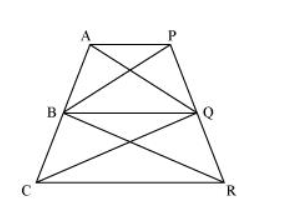# In the given figure, AP || BQ || CR.

Question. In the given figure, $A P\|B Q\| C R .$ Prove that $\operatorname{ar}(A Q C)=\operatorname{ar}(P B R) .$

Solution:Since $\triangle A B Q$ and $\triangle P B Q$ lie on the same base $B Q$ and are between the same parallels $A P$ and $B Q$,

$\therefore$ Area $(\triangle A B Q)=$ Area $(\triangle P B Q) \ldots(1)$

Again, $\triangle B C Q$ and $\triangle B R Q$ lie on the same base $B Q$ and are between the same parallels $B Q$ and $C R$.

$\therefore$ Area $(\Delta B C Q)=$ Area $(\Delta B R Q) \ldots(2)$

On adding equations (1) and (2), we obtain

Area $(\Delta \mathrm{ABQ})+$ Area $(\Delta \mathrm{BCQ})=$ Area $(\Delta \mathrm{PBQ})+$ Area $(\Delta \mathrm{BRQ})$

$\Rightarrow$ Area $(\triangle A Q C)=$ Area $(\triangle P B R)$